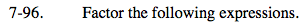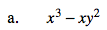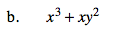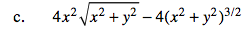### Home > PC > Chapter 7 > Lesson 7.3.1 > Problem7-96

7-96.Factor an 'x' out first. Then factor again.Factor an 'x' out first. Can you factor again?

You cannot factor again. This is NOT a difference of two squares.Change the square root part of the first term to exponential notation.

Factor out 4(x2 + y2)1/2.

Distribute the negative.

Simplify.

$4x^{2}(x^{2}+y^{2})^{1/2}-4(x^{2}+y^{2})^{3/2}$

$4(x^{2}+y^{2})^{1/2}(x^{2}-(x^{2}+y^{2}))$

$4(x^{2}+y^{2})^{1/2}(x^{2}-x^{2}-y^{2})$